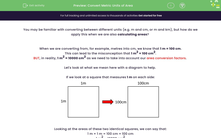# Convert Metric Units of Area

In this worksheet, students will practise converting areas into alternative units of measurement by applying the area conversion factor.Key stage:  KS 4

Year:  GCSE

GCSE Subjects:   Maths

GCSE Boards:   AQA, Eduqas, Pearson Edexcel, OCR,

Popular topics:   Area worksheets, Geometry worksheets

Difficulty level:#### Worksheet Overview

You may be familiar with converting between different units (e.g. m and cm, or m and km), but how do we apply this when we are also calculating areas

When we are converting from, for example, metres into cm, we know that 1 m = 100 cm.

This can lead to the misconception that 1 m2 = 100 cm2.

BUT, in reality, 1 m2 = 10000 cm2 as we need to take into account our area conversion factors

Let's look at what we mean here with a diagram to help.

If we look at a square that measures 1 m on each side:Looking at the areas of these two identical squares, we can say that:

1 m × 1 m = 100 cm × 100 cm

1 m2 = 10000 cm2

Is there a trick?

In a way, yes.

All we need to do for an area conversion is to find the conversion if it was a length, and then square it to get the area conversion.

Let's look at an example to see this in action.

e.g. Convert 1.4 m2 into cm2.

We know that for length, 1 m = 100 cm.

So for area, 1 m2 = 10000 cm2.

So 1.4 m2 = 1.4 × 10000 = 14000 cm2.

e.g. Convert 350 m2 into km2.

We know that for a length, 1 km = 1000 m.

So for area, 1 km2 = 1000000 m2.

So 350 m2 = 350 ÷ 1000000 = 0.00035 m2.

In this activity, we will convert areas (expressed in cm2, m2 and km2) into alternative units of measurement by applying the area conversion factor using the methods shown above.

### What is EdPlace?

We're your National Curriculum aligned online education content provider helping each child succeed in English, maths and science from year 1 to GCSE. With an EdPlace account you’ll be able to track and measure progress, helping each child achieve their best. We build confidence and attainment by personalising each child’s learning at a level that suits them.

Get started